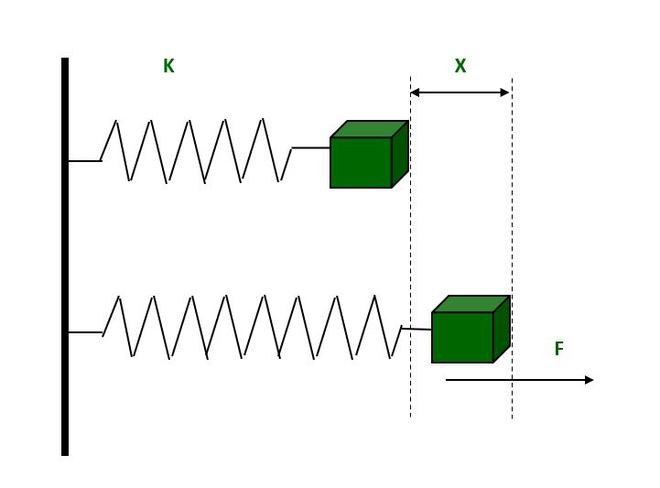GFG App
Open AppBrowser
Continue

Simple harmonic motion, or SHM, is a fascinating type of motion. It is commonly used in the oscillatory motion of objects. SHM is commonly found in springs. Springs have inherent “spring constants” that define their stiffness. Hooke’s law is a well-known law that explains the SHM and provides a formula for the applied force using the spring constant.

### Hooke’s Law

According to Hooke’s law, the force required to compress or extend a spring is proportional to the length stretched. When spring is pulled, Newton’s Third Law of Motion states that it returns with a restoring force. This restoring force follows Hooke’s Law, which relates spring force to constant spring force.Spring Force = -(Spring Constant) × (Displacement)

F = -KX

The negative sign indicates that the reaction force is pointing in the opposite direction.

Where,

F: The restoring force of the spring, directed towards equilibrium.

K: The spring constant in N.m-1.

X: The spring’s displacement from its equilibrium position.

### Spring Constant (K)

The Spring constant is now defined as the force needed per unit of spring extension. Knowing the spring constant allows to easily calculate how much force is required to deform the spring.

From Hooke’s law,

F = -KX

K = -F/ X ⇢ (1)

Equation (1) is a formula for spring constant and It is measured in N/m (Newton per meter).

Spring Constant Dimensional Formula

As known,

F = -KX

Therefore, K = -F/ X

Dimension of F = [MLT-2]

Dimension of X = [L]

Therefore, dimension of K = [MLT−2]/[L] = [MT−2].

### Potential Energy of a Spring (P.E.)

The energy stored in a compressible or stretchable object is referred to as spring potential energy. it is also called Elastic potential energy. It is equal to the force multiplied by the distance traveled.

It is known that, Potential energy = force × displacement

And also force of the spring is equal to the spring constant × displacement. So,

P.E. = 1/2 KX2 .⇢ (2)

The above equation is formula of spring potential energy.

Limitations of Hooke’s law

Hooke’s Law has a limitation in that it is only applicable under the elastic limit of any material, which means that material must be perfectly elastic in order to obey Hooke’s Law. Hooke’s law essentially breaks down beyond the elastic limit.

Applications of Hooke’s Law

• Due to the elasticity of springs, Hooke’s Law is most commonly applied in spring.
• They are used not only in the field of engineering but also in the field of medical science.
• It is used in the lungs, the skin, spring beds, diving boards, and automobile suspension systems.
• It is the fundamental principle underlying the manometer, spring scale, and clock balance wheel.
• It is also the basis for seismology, acoustics, and molecular mechanics.

The following are the drawbacks of Hooke’s Law:

• Hooke’s Law is only applicable in the elastic region after that it fails.
• Hooke’s Law yields accurate results only for solid bodies with small forces and deformations.
• Hooke’s Law is not a general rule.

### Sample Problems

Question 1: What is the definition of the Spring Constant?

When a spring is stretched, the force exerted is proportional to the increase in length from the equilibrium length, according to Hooke’s Law. The spring constant can be calculated using the following formula: k = -F/x, where k is the spring constant. F denotes the force, and x denotes the change in spring length.

Question 2: How Does the Length Affect the Spring Constant?

Assume there is a 6 cm spring with a spring constant k. What happens if the spring is divided into two equal-sized pieces? One of these shorter springs will have a new spring constant of 2k. In general, assuming a specific material spring and thickness, the spring constant of a spring is inversely proportional to the length of the spring.

So, in the preceding example, suppose the spring exactly cut in half, resulting in two shorter springs, each 3 cm in length. For the smaller springs, a spring constant twice as large as the original will be used. This happens because it is inversely proportional to both the spring constant and the spring length.

Question 3: A spring is stretched with a force of 2N by 4 m. Determine its spring constant.

Solution :

Given,

Force, F = 2 N and

Displacement, X = 4 m.

We know that,

The Spring constant, K = – F/X

K = – 2N / 4m

K = – 0.5 Nm-1 .

Question 4:  10 N force is applied to a string and it gets stretched. if the spring constant is 4 Nm-1 then calculate the displacement of the string.

Solution:

Given,

Force, F = 10 N and

Spring constant, K = 4 Nm-1

We know that, F = – KX

X (Displacement) = – F/K

X = – ( 10 N / 4 Nm-1 )

X = – 2.5 m.

Question 5:  How much force is required to stretch a 3-meter spring to 5 meters if the spring constant is 0.1 Nm-1.

Solution :

Given,

Length of spring  = 3m

Spring constant, K = 0.1 Nm-1

Stretch it to 5 meter so the displacement of the spring is  X = 5 – 3 = 2m

Now, Required Force is F = -KX

F = – (0.1 Nm-1 × 2m )

F = – 0.2 N.

My Personal Notes arrow_drop_up